Courses

# Second Law Of Thermodynamics NAT Level - 2

## 10 Questions MCQ Test Topic wise Tests for IIT JAM Physics | Second Law Of Thermodynamics NAT Level - 2

Description
This mock test of Second Law Of Thermodynamics NAT Level - 2 for IIT JAM helps you for every IIT JAM entrance exam. This contains 10 Multiple Choice Questions for IIT JAM Second Law Of Thermodynamics NAT Level - 2 (mcq) to study with solutions a complete question bank. The solved questions answers in this Second Law Of Thermodynamics NAT Level - 2 quiz give you a good mix of easy questions and tough questions. IIT JAM students definitely take this Second Law Of Thermodynamics NAT Level - 2 exercise for a better result in the exam. You can find other Second Law Of Thermodynamics NAT Level - 2 extra questions, long questions & short questions for IIT JAM on EduRev as well by searching above.
*Answer can only contain numeric values
QUESTION: 1

### To make some ice, a freezer extracts 185kJ of heat at –12ºC. The freezer has a coefficient of performance of 5.70. The room temperature is 26ºC. How much heat is delivered to the room (in kJ)

Solution:

To obtain the heat that is delivered to the room, first we have to find out the required work W to run the freezer.
To obtain the required work W to run the freezer,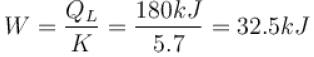Now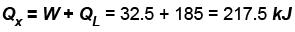⇒ heat delivered to the room would be 217.5kJ

*Answer can only contain numeric values
QUESTION: 2

### An electric current of 3A flows through a resistance of 10Ω. It is being cooled by running water and is kept at temperature 300K, what is the change in entropy per second of the resistance (in Joules degree).

Solution:

There is no change in the entropy of the resistance because there is no change in the state of the wire.

*Answer can only contain numeric values
QUESTION: 3

### Steam at 100ºC is mixed with 1500gms of water at 15ºC so that the final temperature of the mixture is 80ºC the mass of steam is (in gms).

Solution:

Hence, m1 = ?, m2 = 1500gm
T1 = 373K, T2 = 273 + 15 = 288 K
Final temperature of mixture be T = 353 K
m1 × 540 + m1 (373 – 353) = 1500(353 – 288)
(540 + 20)m1 = 1500 × 65
m1 = 175g

*Answer can only contain numeric values
QUESTION: 4

A refrigerator is such that it extracts 20kJ of heat at a lower temperature and give out 50kJ of heat to the surrounding, what is the coefficient of performance (K)

Solution: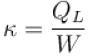W = QK – QL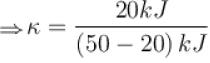= 20/30
K = 0.67

*Answer can only contain numeric values
QUESTION: 5

The two ends of a rod of thermal conductivity K, length L and cross-section A are immersed in two heat reservoirs at temp T1 = 800K and T2 = 500K. The rod is thermally insulated from the surroundings. In a steady state, the entropy of the universe in per unit time at the rate of (in units of kA/L)

Solution:

Since the rod is thermally insulated from the surrounding and its two ends are immersed in two heat reservoirs. then,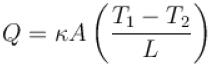where K , is thermal conductivity.
Now, increase in entropy is given by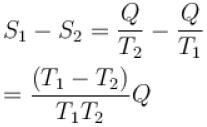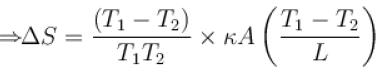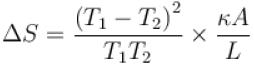T1 = 800K, T2 = 500K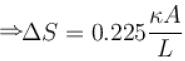*Answer can only contain numeric values
QUESTION: 6

1000gm of water at temperature 300K is isobarically and adiabatically mixed with an equal mass of water at 500K. Cp = 0.01 cal K-1gm-1 . The entropy change of the universe in (cal K-1 ) is

Solution: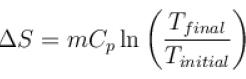Now,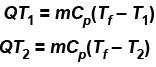Also, the higher temperature loses heat and water temperature gains heat, we can write,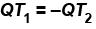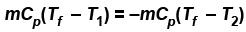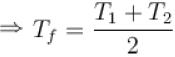Since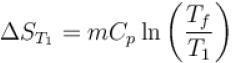and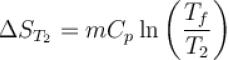Total entropy change of universe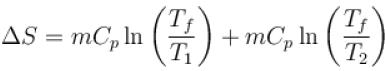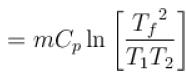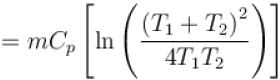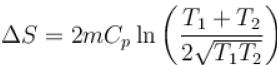m = 1000gm, CP = 0.01Cal K-1 gm-1
T1 = 300K, T2 = 500K
⇒ ΔS = 0.645

*Answer can only contain numeric values
QUESTION: 7

Engine A, compared to engine B, produces, per cycle, five times the work but receives three times the heat input and exhaust out twice the heat. Determine the efficiency of engine B

Solution:

Let work done by the engine B is WB.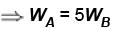Let heat input of engine B is Qi,B.
⇒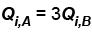Let heat output of engine B is Q0,B
⇒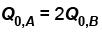Work done in an engine is equal to the difference of input heat and output heat.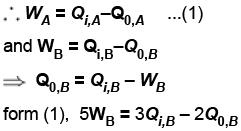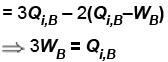⇒ Efficiency of engine B will be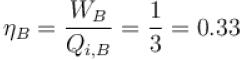*Answer can only contain numeric values
QUESTION: 8

At what temperature in Kelvin scale, fluid are assumed to have zero entropy

Solution:

S = KblnΩ
At 0 K, we know the system is certainly in the ground state Ω = 1, so the definition returns zero.

*Answer can only contain numeric values
QUESTION: 9

A mixture of 1.78kg of water and 262g of ice at 0ºC is, in a reversible process, brought to a final equilibrium state where the water/ice ratio, by mass is 1:1 at 0ºC. Calculate the entropy change of the system during this process (in J/K). L = 333 × 103 J/kg for water

Solution:

Mass of water = 1.78kg
Mass of ice = 262g
Mass of ice-water mixture = 1.78 kg + 262g
= 2.04kg
If eventually, ice and and water have the same mass, then the final state will have 1.02kg of each.
Thus, mass of water that changed into ice m will be the difference of mass of water mW and mass of final state mS .
So, m = mW – mS
⇒m = 1.78 – 1.02
= 0.76kg
The change of water at 0ºC to ice at 0ºC is isothermal.
⇒ ΔS = –mL/T
= –(0.76kg) (333×103J/kg) (273K)
= –927 J/K
⇒ Change in entropy of the system during this process will be –927J/K.

*Answer can only contain numeric values
QUESTION: 10

An oil bath kept at 27ºC is being supplied heat at the rate of 100Js-1 . Assuming the process to be quasi-static, the rate of increase of entropy of the system is approximately(in JK-1 s-1 )?

Solution:

Change in entropy =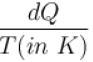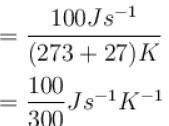= 0.33Js-1K-1